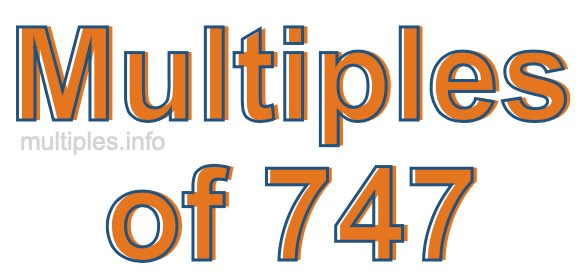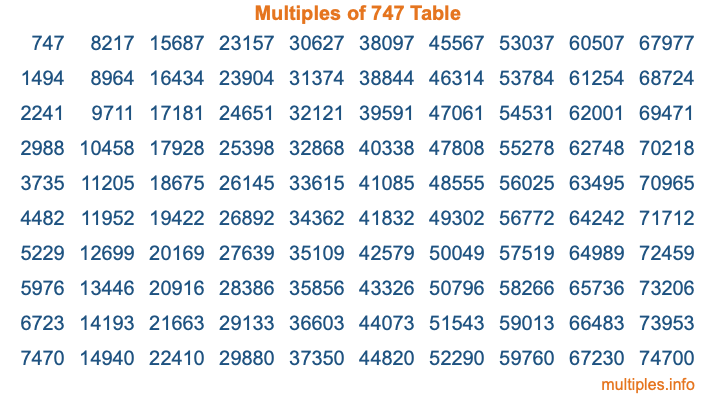Multiples of 747Welcome to the Multiples of 747 page. Here we will first teach you everything you will ever need to know about the multiples of 747, and then give you a study guide summary of everything we taught you to make sure you remember it all. Use this page to look up facts and learn information about the multiples of 747. This page will make you a multiples of seven hundred forty-seven expert!

Definition of Multiples of 747
Multiples of 747 are all the numbers that when divided by 747 equal an integer. Each of the multiples of 747 are called a multiple. A multiple of 747 is created by multiplying 747 by an integer.

Therefore, to create a list of multiples of 747, you start with 1 multiplied by 747, then 2 multiplied by 747, then 3 multiplied by 747, and so on for as long as you want. Thus, the list of the first five multiples of 747 is 747, 1494, 2241, 2988, and 3735. To see a larger list of multiples of 747, see the printable image of Multiples of 747 further down on this page. We also have a category where you can choose any nth multiple of 747.

Multiples of 747 Checker
The Multiples of 747 Checker below checks to see if any number of your choice is a multiple of 747. In other words, it checks to see if there is any number (integer) that when multiplied by 747 will equal your number. To do that, we divide your number by 747. If the the quotient is an integer, then your number is a multiple of 747.

Is  a multiple of 747?

Least Common Multiple of 747 and ...
A Least Common Multiple (LCM) is the lowest multiple that two or more numbers have in common. This is also called the smallest common multiple or lowest common multiple and is useful to know when you are adding our subtracting fractions. Enter one or more numbers below (747 is already entered) to find the LCM.

Check out our LCM Calculator if you need more details about the Least Common Multiple or if you need the LCM for different numbers for adding and subtraction fractions.

nth Multiple of 747
As we stated above, 747 is the first multiple of 747, 1494 is the second multiple of 747, 2241 is the third multiple of 747, and so on. Enter a number below to find the nth multiple of 747.

th multiple of 747

Multiples of 747 vs Factors of 747
747 is a multiple of 747 and a factor of 747, but that is where the similarities end. All postive multiples of 747 are 747 or greater than 747. All positive factors of 747 are 747 or less than 747.

Below is the beginning list of multiples of 747 and the factors of 747 so you can compare:

Multiples of 747: 747, 1494, 2241, 2988, 3735, etc.

Factors of 747: 1, 3, 9, 83, 249, 747

As you can see, the multiples of 747 are all the numbers that you can divide by 747 to get a whole number. The factors of 747, on the other hand, are all the whole numbers that you can multiply by another whole number to get 747.

It's also interesting to note that if a number (x) is a factor of 747, then 747 will also be a multiple of that number (x).

Multiples of 747 vs Divisors of 747
The divisors of 747 are all the integers that 747 can be divided by evenly. Below is a list of the divisors of 747.

Divisors of 747: 1, 3, 9, 83, 249, 747

The interesting thing to note here is that if you take any multiple of 747 and divide it by a divisor of 747, you will see that the quotient is an integer.

Multiples of 747 Table
Below is an image of the first 100 multiples of 747 in a table. The table is in chronological order, column by column. The first column has the first ten multiples of 747, the second column has the next ten multiples of 747, and so on.The Multiples of 747 Table is also referred to as the 747 Times Table or Times Table of 747. You are welcome to print out our table for your studies.

Negative Multiples of 747
Although not often discussed or needed in math, it is worth mentioning that you can make a list of negative multiples of 747 by multiplying 747 by -1, then by -2, then by -3, and so on, to get the following list of negative multiples of 747:

-747, -1494, -2241, -2988, -3735, etc.

Multiples of 747 Summary
Below is a summary of important Multiples of 747 facts that we have discussed on this page. To retain the knowledge on this page, we recommend that you read through the summary and explain to yourself or a study partner why they hold true.

There are an infinite number of multiples of 747.

A multiple of 747 divided by 747 will equal a whole number.

747 divided by a factor of 747 equals a divisor of 747.

The nth multiple of 747 is n times 747.

The largest factor of 747 is equal to the first positive multiple of 747.

747 is a multiple of every factor of 747.

747 is a multiple of 747.

A multiple of 747 divided by a divisor of 747 equals an integer.

747 divided by a divisor of 747 equals a factor of 747.

Any integer times 747 will equal a multiple of 747.

Multiples of a Number
Here you can get the multiples of another number, all with the same attention to detail as we did for multiples of 747 on this page.

Multiples of
Multiples of 748
Did you find our page about multiples of seven hundred forty-seven educational? Do you want more knowledge? Check out the multiples of the next number on our list!